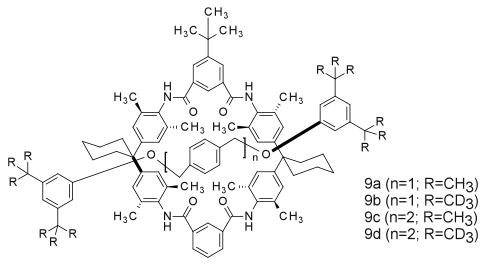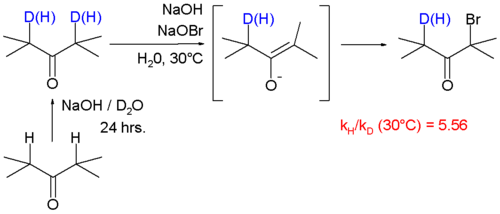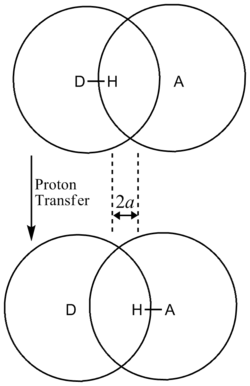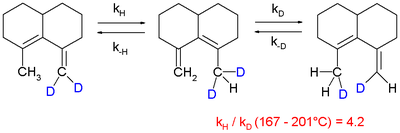# Kinetic isotope effect

﻿
Kinetic isotope effect

The kinetic isotope effect (KIE) is the ratio of reaction rates of two different isotopically labeled molecules in a chemical reaction. It is also called "isotope fractionation," although this term is somewhat broader in meaning. A KIE involving hydrogen and deuterium is represented as:$KIE = \frac{k_H}{k_D} \qquad \qquad (1)$

with kH and kD are reaction rate constants.

An isotopic substitution can greatly modify the reaction rate when the isotopic replacement is in a chemical bond that is broken or formed in the rate limiting step. In such a case, the change is termed a primary isotope effect. When the substitution is not involved in the bond that is breaking or forming, a smaller rate change, termed a secondary isotope effect is observed. Thus, the magnitude of the kinetic isotope effect can be used to elucidate the reaction mechanism. If other steps are partially rate-determining, the effect of isotopic substitution will be masked. This masking of the intrinsic isotope effect has been referred to as 'kinetic complexity'.

Isotopic rate changes are most pronounced when the relative mass change is greatest, since the effect is related to vibrational frequencies of the affected bonds. For instance, changing a hydrogen atom to deuterium represents a 100% increase in mass, whereas in replacing carbon-12 with carbon-13, the mass increases by only 8%. The rate of a reaction involving a C-H bond is typically 6 to 10 times faster than the corresponding C-D bond, whereas a 12C reaction is only ~1.04 times faster than the corresponding 13C reaction (even though, in both cases, the isotope is one atomic mass unit heavier).

Isotopic substitution can modify the rate of reaction in a variety of ways. In many cases, the rate difference can be rationalized by noting that the mass of an atom affects the vibrational frequency of the chemical bond that it forms, even if the electron configuration is nearly identical. Heavier atoms will (classically) lead to lower vibration frequencies, or, viewed quantum mechanically, will have lower zero-point energy. With a lower zero-point energy, more energy must be supplied to break the bond, resulting in a higher activation energy for bond cleavage, which in turn lowers the measured rate (see, for example, the Arrhenius equation).

The Swain equation (also cited as the Swain-Schaad-Stivers equations) relate the intrinsic KIEs for the hydrogen isotopes to each other - i.e. using these relationships, one can extract the protium/tritium (H/T) KIE or the deuterium/tritium (D/T) KIE from the protium/deuterium KIE (H/D); similarly, if one of these KIEs is known, the other two can be extracted using the appropriate form of the Swain-Schaad equation.

The kinetic isotope effect leads to a specific distribution of deuterium isotopes in natural products, depending on the route they were synthesized in nature. By NMR spectroscopy, it is therefore easy to detect whether the alcohol in wine was fermented from glucose, or from illicitly added saccharose.

Isotopic effect expressed with Eq. (1) only refer to reactions that can be described with first-order kinetics. In all instances in which this is not possible, transient kinetic isotope effects should be taken into account using the GEBIK and GEBIF equations.

## Inverse KIE's

Reactions are known where the deuterated species reacts faster than the undeuterated analogue, and these cases are said to exhibit inverse kinetic isotope effects (IKIE). IKIE's are often observed in the reductive elimination of alkyl metal hydrides, e.g. (Me2NCH2CH2NMe2)PtMe(H). In such cases the C-D bond in the transition state, an agostic species, is highly stabilized relative to the C-H bond.

## Mathematical details in a diatomic molecule

One approach to studying the effect is for that of a diatomic molecule. The fundamental vibrational frequency (ν) of a chemical bond between atom A and B is, when approximated by a harmonic oscillator:$\nu = \frac{1}{2 \pi} \sqrt{\frac{k}{\mu}}$

where k is the spring constant for the bond, and μ is the reduced mass of the A-B system:$\mu = \frac{m_A m_B}{m_A + m_B}$

(mi is the mass of atom i). Quantum mechanically, the energy of the n-th level of a harmonic oscillator is given by:$E_n = h \nu \left ( n + \frac{1}{2} \right )$

Thus, the zero-point energy (n = 0) will decrease as the reduced mass increases. With a lower zero-point energy, more energy is required to overcome the activation energy for bond cleavage.

In changing a carbon-hydrogen bond to a carbon-deuterium bond, k remains unchanged, but the reduced mass µ is different. As a good approximation, on going from C-H to C-D, the reduced mass increases by a factor of approximately 2. Thus, the frequency for a C-D bond should be approximately 1/√2 or 0.71 times that of the corresponding C-H bond. This effect is much larger than for changing the carbon-12 to carbon-13.

## Secondary isotope effect

The secondary kinetic isotope effect (SKIE) arises in cases where the isotopic substitution is remote from the bond being broken. The remote atom, nonetheless, influences the internal vibrations of the system that via changes in the zero point energy (ZPE) affect the rates of chemical reactions. Such effects are expressed as ratios of rate for the light isotope to that of the heavy isotope and can be "normal" (ratio is greater than or equal to 1) or "inverse" (ratio is less than 1) effects. SKIE are defined as α,β (etc.) secondary isotope effects where such prefixes refer to the position of the isotopic substitution relative to the reaction center (see alpha and beta carbon). The prefix α refers to the isotope associated with the reaction center while the prefix β refers to the isotope associated with an atom neighboring the reaction center and so on.

In physical organic chemistry, SKIE is discussed in terms of electronic effects such as induction, bond hybridization, or hyperconjugation. These properties are determined by electron distribution, and depend upon vibrationally averaged bond length and angles that are not greatly affected by isotopic substitution. Thus, the use of the term "electronic isotope effect" while legitimate is discouraged from use as it can be misinterpreted to suggest that the isotope effect is electronic in nature rather than vibrational.

SKIE's can be explained in terms of changes in orbital hybridization. When the hybridization of a carbon atom changes from sp3 to sp2, a number of vibrational modes (stretches, in-plane and out-of-plane bending) are affected. The in-plane and out-of-plane bending in an sp3 hybridized carbon are similar in frequency due to the symmetry of an sp3 hybridized carbon. In an sp2 hybridized carbon the in-plane bend is much stiffer than the out-of-plane bending resulting in a large difference in the frequency, the ZPE and thus the SKIE (which exists when there is a difference in the ZPE of the reactant and transition state). The theoretical maximum change caused by the bending frequency difference has been calculated as 1.4.

When carbon undergoes a reaction that changes its hybridization from sp3 to sp2, the out of plane bending force constant at the transition state is weaker as it is developing sp2 character and a "normal" SKIE is observed with typical values of 1.1 to 1.2. Conversely, when carbon's hybridization changes from sp2 to sp3, the out of plane bending force constants at the transition state increase and an inverse SKIE is observed with typical values of 0.8 to 0.9.

More generally the SKIE for reversible reactions can be "normal" one way and "inverse" the other if bonding in the transition state is midway in stiffness between substrate and product, or they can be "normal" both ways if bonding is weaker in the transition state, or "inverse" both ways if bonding is stronger in the transition state than in either reactant.

An example of an "inverse" α secondary kinetic isotope effect can be seen in the work of Fitzpatrick and Kurtz who used such an effect to distinguish between two proposed pathways for the reaction of d-amino acid oxidase with nitroalkane anions. Path A involved a nucleophilic attack on the coenzyme FAD, while path B involves a free-radical intermediate. As path A results in the intermediate carbon changing hybridization from sp2 to sp3 an "inverse" a SKIE is expected. If path B occurs then no SKIE should be observed as the free radical intermediate does not change hybridization. An SKIE of 0.84 was observed and Path A verified as shown in the scheme below.Another example of an SKIE is the oxidation of benzyl alcohols by dimethyldioxirane where three transition states for different mechanisms were proposed. Angelis et al. used SKIE to show that TS| was the transition state as TS|| and TS||| should show a large SKIE due to the hyperconjugative effect of the five hydrogen atoms in 6-d0 versus the five deuterium atoms in 6-d5 (kH/kD = 1.03–1.1 per deuterium atom). This did not match the observed SKIE of approximately 1 which eliminated TS|| and TS|||.## Steric isotope effect

The steric isotope effect is an SKIE that does not involve bond breaking or formation. This effect is attributed to the different vibrational amplitudes of isotopologues (molecular entities differing in isotopic composition only.) An example of such an effect can be seen in the work of Kurt Mislow et al. who demonstrated through the racemization of 9,10-dihydro-4,5-dimethylphenanthrene that the smaller amplitude of vibration for deuterium as compared to hydrogen in C-H (Carbon -Hydrogen), C-D (Carbon -Deuterium) bonds results in a smaller van der Waals radius or effective size in addition to a difference in the ZPE between the two. When there is a greater effective bulk of molecules containing one over the other this may be manifested by a steric effect on the rate constant. For the example above deuterium racemizes faster than the hydrogen isotopologue resulting in a steric isotope effect.

Another example of the steric isotope effect has been reported by Schalley and Felder in the deslipping reaction of rotaxanes where the deuterium isotope due to its smaller effective size allows faster passage through the stoppers resulting in faster rates of deslipping for the deuterated rotaxanes. A depiction of the deslippage reaction of rotaxanes where the mechanical bond is broken and the components freed without cleavage of chemical bonds.## Applications

Reaction mechanisms are routinely elucidated using the kinetic isotope effect, e.g. the halogenation of toluene:In this particular "intramolecular KIE" study the radical substitution of hydrogen by bromine is examined in the reaction involving N-bromosuccinimide as the brominating agent. With mono-deuterated toluene (obtained by organic reduction of benzyl chloride with zinc and deuterated acetic acid), it was found that hydrogen is replaced by bromine faster than deuterium, the reaction product gets enriched in deuterium. A KIE of 4.86 was determined. This finding is in accordance with the general accepted view of a radical substitution in which a hydrogen atom is removed by a bromine free radical species in the rate-determining step.

A large KIE of 5.56 is also reported for reaction of ketones with bromine and sodium hydroxide forming a haloketone with the α-carbonyl positions deuterated.In this reaction the rate-limiting step is enolate formation by proton (deuterium) abstraction from the ketone by base. In this study the KIE is calculated from the reaction rate constants for regular 2,4-dimethyl-3-pentanone and its deuterated isomer by optical density measurements.

## Tunneling

In some cases, an additional rate enhancement is seen for the lighter isotope, possibly due to quantum mechanical tunnelling. This is typically only observed for reactions involving bonds to hydrogen atoms. Tunneling occurs when a molecule penetrates through a potential energy barrier rather than over it. Although not allowed by the laws of classical mechanics, particles can pass through classically forbidden regions of space in quantum mechanics based on wave-particle duality.The potential energy well of a tunneling reaction. The dash-red arrow shows the classical activated process, while the red arrow shows the tunneling path.

Analysis of tunneling can be made using Bell’s modification of the Arrhenius equation which includes the addition of a tunneling factor, Q:

k = QAe E / RT

where A is the Arrhenius parameter, E is the barrier height and$Q = \frac{e^\alpha}{\beta-\alpha}(\beta e^{-\alpha}-\alpha e^{- \beta})$

where$\alpha=\frac{E}{RT}$

and$\beta=\frac{2a \pi ^2(2mE)^{1/2}}{h}$

Examination of the β term shows exponential dependency on the mass of the particle. As a result, tunneling is much more likely for a lighter particle such as hydrogen. Simply doubling the mass of a tunneling proton by replacing it with its deuterium isotope drastically reduces the rate of such reactions. As a result, very large kinetic isotope effects are observed that can not be accounted for by differences in zero point energies.Donor-Acceptor Model of a proton transfer.

In addition, the β term depends linearly with barrier width, 2a. As with mass, tunneling is greatest for small barrier widths. Optimal tunneling distances of protons between donor and acceptor atom is 0.4 Å.

### Temperature Dependence in Tunneling

Tunneling is a quantum mechanical effect tied to the laws of wave mechanics, not kinetics. Therefore, tunneling tends to become more important at low temperatures, where even the smallest kinetic energy barriers may not be overcome but can be tunneled through.

Peter S. Zuev et al. reported rate constants for the ring expansion of 1-methylcyclobutylfluorocarbene to be 4.0 x 10−6/s in nitrogen and 4.0 x 10−5/s in argon at 8 kelvin. They calculated that at 8 kelvin, the reaction would proceed via a single quantum state of the reactant so that the reported rate constant is temperature independent and the tunneling contribution to the rate was 152 orders of magnitude greater than the contribution of passage over the transition state energy barrier.

So despite the fact that conventional chemical reactions tend to slow down dramatically as the temperature is lowered, tunneling reactions rarely change at all. Particles that tunnel through an activation barrier are a direct result of the fact that the wave function of an intermediate species, reactant or product is not confined to the energy well of a particular trough along the energy surface of a reaction but can “leak out” into the next energy minimum. In light of this, tunneling should be temperature independent.

Noboru Fujisaki et al. reported the gas phase H/D kinetic isotope effect for the hydrogen abstraction from some n-alkanes and cycloalkanes by hydrogen atoms over the temperature range 363-463 K. The data they obtained was characterized by small preexponential factor ratios AH/AD ranging from 0.43 to 0.54 and large activation energy differences from 9.0 to 9.7 kJ/mol. Basing their arguments on transition state theory, the small A factor ratios associated with the large activation energy differences (usually about 4.5 kJ/mol for C-H(D) bonds) provided strong evidence for tunneling. For the purpose of this discussion, what is important to note is that the A factor ratio for the various paraffins they used was approximately constant throughout the temperature range.

The observed fact that tunneling isn't entirely temperature independent can be explained by the fact that not all molecules of a certain species occupy their vibrational ground state at varying temperatures. Adding thermal energy to a potential energy well could cause higher vibrational levels other than the ground state to become populated. For a conventional kinetically driven reaction, this excitation would only have a small influence on the rate. However, for a tunneling reaction, the difference between the zero point energy and the first vibrational energy level could be huge. The tunneling correction term Q is linearly dependent on barrier width and this width is significantly diminished as the number vibrational modes on the Morse potential increase. The decrease of the barrier width can have such a huge impact on the tunneling rate that even a small population of excited vibrational states would dominate this process.

### Criteria for KIE tunneling

To determine if tunneling is involved in KIE of a reaction with H or D, there are a few criteria:
1.Δ(EaH-EaD) > Δ(ZPEH-ZPED) (Ea=activation energy; ZPE=zero point energy)
2.Reaction still proceeds at lower temperatures.
3.The Arrhenius pre-exponential factors AD/AH is not equal to 1.
4.There is a large negative entropy of activation.
5.The geometries of the reactants and products are usually very similar.

Also for reactions where isotopes include H, D and T, a criterion of tunneling is the Swain-Schaad relations which compare the rate constants(k) of the reactions when H,D or T are the protons: kH/kT=(kD/kT)X and kH/kT=(kH/kD)Y
Experimental values of X exceeding 3.26 and Y lower than 1.44 are evidence of a certain amount of contribution from tunneling.

### Examples for tunneling in KIE

In organic reactions, this proton tunneling effect has been observed in such reactions as the deprotonation and iodination of nitropropane with hindered pyridine base with a reported KIE of 25 at 25 °C:and in a 1,5-sigmatropic hydrogen shift although it is observed that it is difficult to extrapolate experimental values obtained at elevated temperatures to lower temperatures:There has long been speculation that high efficiency of enzyme catalysis in proton or hydride ion transfer reactions could be due partly to the quantum mechanical tunneling effect. Environment at the active site of an enzyme positions the donor and acceptor atom close to the optimal tunneling distance, where the amino acid side chains can "force" the donor and acceptor atom closer together by electrostatic and noncovalent interactions. It is also possible that the enzyme and its unusual hydrophobic environment inside a reaction site provides tunneling-promoting vibration. Studies on ketosteroid isomerase have provided experimental evidence that the enzyme actually enhances the coupled motion/hydrogen tunneling by comparing primary and secondary kinetic isotope effects of the reaction under enzyme catalyzed and non-enzyme catalyzed conditions.

There are many examples of proton tunneling in enzyme catalyzed reactions that were discovered by KIE. A well studied example is methylamine dehydrogenase, where large primary KIEs of 5-55 have been observed for the proton transfer step.Mechanism of methylamine dehydrogenase, the quinoprotein converts primary amines to aldehyde and ammonia.

Another example of tunneling contribution to proton transfer in enzymatic reactions is the reaction carried out by alcohol dehydrogenase. Competitive KIEs for the hydrogen transfer step at 25 °C resulted in 3.6 and 10.2 for primary and secondary KIEs, respectively.Mechanism of alcohol dehydrogenase. The rate limiting step is the proton transfer

• Transient kinetic isotope effect
• Isotopic ratio
• Isotopic composition
• Fractionation factor
• Isotopic enrichment
• Magnetic isotope effect

Wikimedia Foundation. 2010.

### Look at other dictionaries:

• Isotope — This article is about the atomic variants of chemical elements. For the British jazz fusion band, see Isotope (band). Isotopes redirects here. For the minor league baseball team, see Albuquerque Isotopes. Isotopes are variants of atoms of a… …   Wikipedia

• Isotope separation — is the process of concentrating specific isotopes of a chemical element by removing other isotopes, for example separating natural uranium into enriched uranium and depleted uranium. This is a crucial process in the manufacture of uranium fuel… …   Wikipedia

• isotope — isotopic /uy seuh top ik/, adj. isotopically, adv. /uy seuh tohp /, n. Chem. any of two or more forms of a chemical element, having the same number of protons in the nucleus, or the same atomic number, but having different numbers of neutrons in… …   Universalium

• Mössbauer effect — Physics. the phenomenon in which an atom in a crystal undergoes no recoil when emitting a gamma ray, giving all the emitted energy to the gamma ray, resulting in a sharply defined wavelength. [1955 60; named after R. MÖSSBAUER] * * * ▪ physics… …   Universalium

• Stable isotope — Graph of isotopes/nuclides by type of decay. Orange and blue nuclides are unstable, with the black squares between these regions representing stable nuclides. The unbroken line passing below many of the nuclides represents the theoretical… …   Wikipedia

• Enzyme kinetics — is the study of the chemical reactions that are catalysed by enzymes, with a focus on their reaction rates. The study of an enzyme s kinetics reveals the catalytic mechanism of this enzyme, its role in metabolism, how its activity is controlled,… …   Wikipedia

• Heavy water — Not to be confused with hard water or tritiated water. Deuterium oxide IUPAC name …   Wikipedia

• Efecto isotópico cinético — Saltar a navegación, búsqueda Para otros usos de este término, véase Efecto isotópico (desambiguación). El efecto isotópico cinético (EIC) es una variación en la velocidad de una reacción química cuando en uno de los reactivos un átomo es… …   Wikipedia Español

• Equilibrium fractionation — Equilibrium isotope fractionation is the partial separation of isotopes between two or more substances in chemical equilibrium. Equilibrium fractionation is strongest at low temperatures, and (along with kinetic isotope effects) forms the basis… …   Wikipedia

• Nuclear chemistry — is the subfield of chemistry dealing with radioactivity, nuclear processes and nuclear properties. It is the chemistry of radioactive elements such as the actinides, radium and radon together with the chemistry associated with equipment (such as… …   Wikipedia